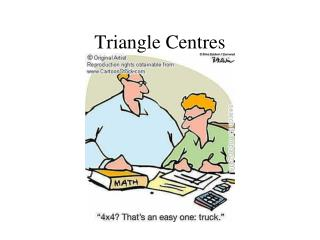DownloadDownload PresentationTriangle Centres

# Triangle Centres

Download Presentation## Triangle Centres

- - - - - - - - - - - - - - - - - - - - - - - - - - - E N D - - - - - - - - - - - - - - - - - - - - - - - - - - -
##### Presentation Transcript

1. Triangle Centres

2. Mental Health Break

3. Given the following triangle, find the: • centroid • orthocenter • circumcenter

4. Centroid • Equation of AD (median) • Strategy…. • Find midpoint D • Find eq’n of AD by • Find slope “m” of AD using A & D • Plug “m” & point A or D into y=mx+b & solve for “b” • Now write eq’n using “m” & “b” Remember – the centroid is useful as the centre of the mass of a triangle – you can balance a triangle on a centroid!

5. Centroid Equation of AD (median)

6. Centroid • Equation of BE (median) • Strategy…. • Find midpoint E • Find eq’n of BE by • Find slope “m” of BE using B & E • Plug “m” & point B or E into y=mx+b & solve for “b” • Now write eq’n using “m” & “b”

7. Centroid Equation of BE (median)

8. Centroid Question? Do we have to find the equation of median CF also?

9. Centroid No We only need the equations of 2 medians… So, what do we do now?

10. Centroid We need to find the Point of Intersection for medians AD & BE using either substitution or elimination

11. Centroid Equation of median AD Equation of median BE

12. Centroid • Equation of AD (median) • Strategy…. • Find midpoint D • Find eq’n of AD by • Find slope “m” of AD using A & D • Plug “m” & point A or D into y=mx+b & solve for “b” • Now write eq’n using “m” & “b”

13. Centroid Eq’n AD – Midpoint of BC

15. Centroid Eq’n AD – Finding “b”

16. Centroid Eq’n AD – Equation

17. Centroid Eq’n BE – Midpoint of AC

18. Centroid Eq’n BE – Slope of BE

19. Centroid Eq’n BE – Finding “b”

20. Centroid Eq’n BE – Equation

21. Centroid – Intersection of Eq’n AD & BE

23. Centroid – Intersection of Eq’n AD & BE Substitute y = 1 into one of the equations Therefore, the point of intersection is (1,1)

24. Orthocentre • Equation of altitude AD • Strategy…. • Find “m” of BC • Take –ve reciprocal of “m” of BC to get “m” of AD • Find eq’n of AD by • Plug “m” from 2. & point A into y=mx+b & solve for “b” • Now write eq’n using “m” & “b”

25. Centroid – Intersection of Eq’n AD & BE Therefore, the Centroid is (1,1)

26. Orthocentre Equation of altitude AD

27. Orthocentre • Equation of altitude BE • Strategy…. • Find “m” of AC • Take –ve reciprocal of “m” of AC to get “m” of BE • Find eq’n of BE by • Plug “m” from 2. & point B into y=mx+b & solve for “b” • Now write eq’n using “m” & “b”

28. Orthocentre Equation of altitude BE

29. Orthocentre Question? Do we have to find the equation of altitude CF also?

30. Orthocentre No We only need the equations of 2 altitudes… So, what do we do now?

31. Orthocentre We need to find the Point of Intersection for altitudes AD & BE using either substitution or elimination

32. Orthocentre Equation of altitude AD Equation of altitude BE

33. Orthocentre Eq’n AD – Slope of BC then Slope of AD

34. Orthocentre Eq’n AD – Finding “b”

35. Orthocentre Eq’n AD – Equation

36. Orthocentre Eq’n BE – Slope of AC then Slope of BE

37. Orthocentre Eq’n BE – Finding “b”

38. Orthocentre Eq’n BE – Equation

39. Orthocentre – Intersection of Eq’n AD & BE

41. Orthocentre – Intersection of Eq’n AD & BE Substitute y = 1 into one of the equations Therefore, the point of intersection or Orthocentre

42. Circumcenter • Equation of ED (perpendicular bisector) • Strategy… (use A (-1, 4), B (-1, -2) & C(5, 1)) • Find midpoint D • Find eq’n of ED by • Find slope “m” of BC using B & E • Take –ve reciprocal to get “m” of ED • Plug “m” ED & point D into y = mx+b & solve for b • Now write eq’n using “m” & “b”

43. Circumcenter Equation of ED (perpendicular bisector)

44. Circumcenter • Equation of FG (perpendicular bisector) • Strategy… (use A (-1, 4), B (-1, -2) & C(5, 1)) • Find midpoint F • Find eq’n of ED by • Find slope “m” of AC using A & C • Take –ve reciprocal to get “m” of FG • Plug “m” FG & point F into y = mx+b & solve for b • Now write eq’n using “m” & “b”

45. Circumcenter Question? Do we have to find the equation of perpendicular bisector HI?

46. Circumcenter No We only need the equations of 2 perpendicular bisectors… So, what do we do now?

47. Circumcenter We need to find the Point of Intersection for perpendicular bisectors ED & FG using either substitution or elimination

48. Circumcenter Equation of perpendicular bisector ED Equation of perpendicular bisector FG

49. Circumcenter Equation of FG (perpendicular bisector)

50. Circumcentre eq’n ED – Midpoint of BC